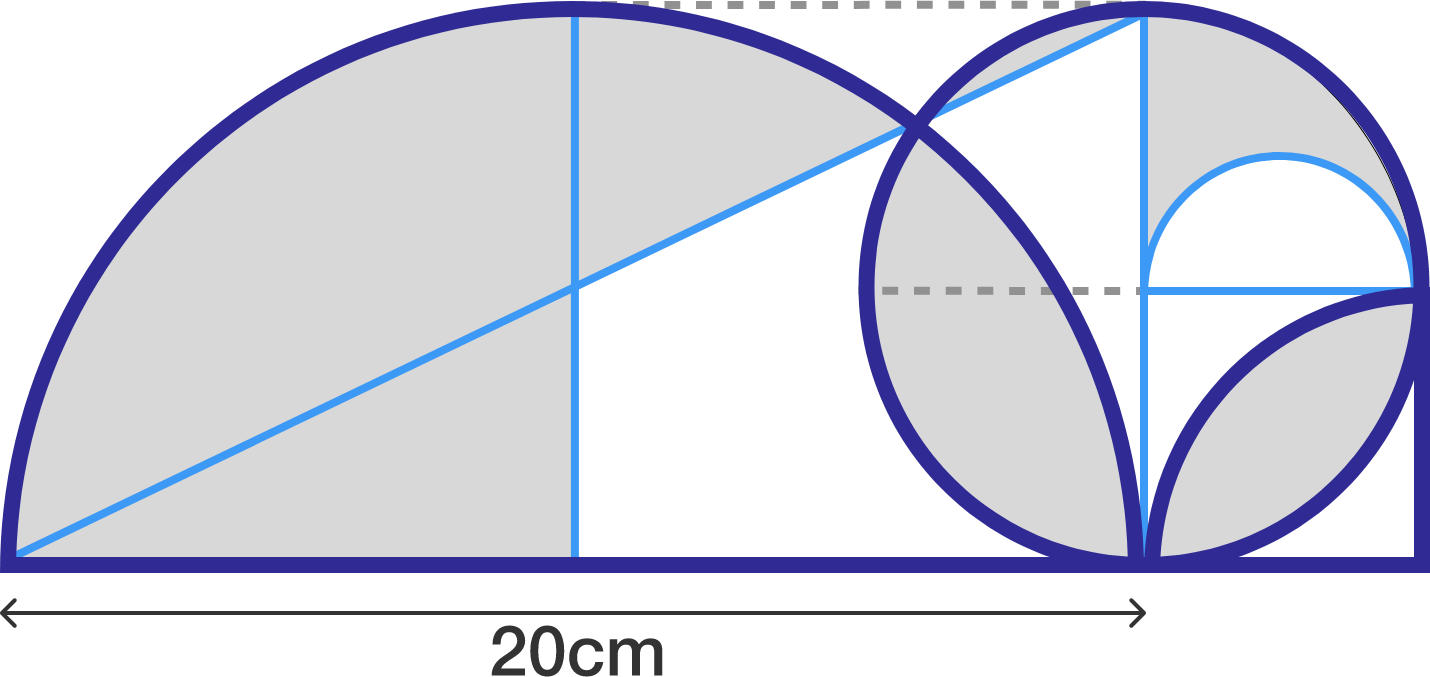# Vicious Circles And Savage Shapes

Geometry Level 1The following figure consists of a large semicircle of diameter $20\text{ cm}$, a smaller full circle of diameter $10\text{ cm}$, a semicircle of diameter $5\text{ cm}$, a quadrant of radius $5 \text{ cm}$, and a right-angled triangle of sides $20\text{ cm}$ and $10\text{ cm}$. Find the shaded area in $\text{cm}^2$.